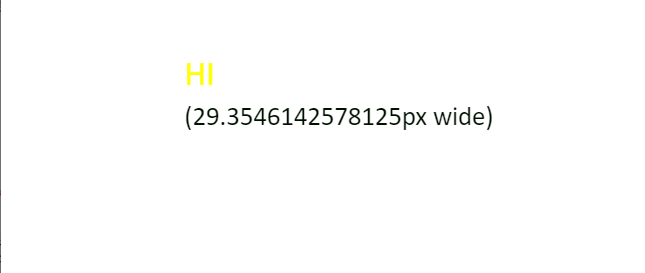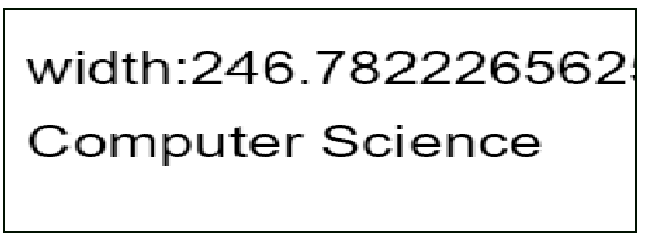# How to find the height of a text in HTML canvas using JavaScript ?

• Difficulty Level : Medium
• Last Updated : 25 Dec, 2020

In this article, we will find the height of the text canvas using JavaScript.

Approach: In the following example, the height attribute of the HTML canvas is used. First set the font in pt to set the height.

`context.font = '26pt Calibri';`

Then the current text is aligned in the center by using values ‘middle’ and color ‘yellow’.

```context.textAlign = 'middle';
context.fillStyle = 'yellow';```

Then, check the width of the text using the measureText() method before writing to the canvas. Finally, the text is written on the canvas using the fillText() method.

Example 1:

## HTML

 ```<``html``>`` ` `<``head``>``    ``<``style``>``        ``body {``            ``margin: 0px;``            ``padding: 0px;``        ``}``    `````` ` `<``body``>``    ``<``canvas` `id``=``"myCanvas"` `width``=``"550"` `height``=``"200"``>``    ````    ``<``script``>``        ``var canvas = document.getElementById('myCanvas');``        ``var context = canvas.getContext('2d');``        ``var x = canvas.width / 3;``        ``var y = canvas.height / 2 - 15;``        ``var text = 'HI ';`` ` `        ``// Set the font to set the height``        ``context.font = '26pt Calibri';``        ``context.textAlign = 'middle';``        ``context.fillStyle = 'yellow';``        ``context.fillText(text, x, y);``        ``context.font = '20pt Calibri';`` ` `        ``// Check the width of the text ``        ``var metrics = context.measureText(text);``        ``var width = metrics.width;`` ` `        ``// Text is aligned in the left      ``        ``context.textAlign = 'left';``        ``context.fillStyle = '#010';``         ` `        ``context.fillText('(' + width ``            ``+ 'px wide)', x, y + 40);``    `````` ` ``

Output:Approach: First get the 2d drawing context of the canvas by using getContext() method. Set the font and the text string. Then write the text with x and y co-ordinates using fillText() method as given below.

Example 2:

## HTML

 ```<``html``>`` ` `<``body``>``    ``<``canvas` `id``=``"Canvas"` `width``=``"300"` `height``=``"150"` `        ``style``=``"border:1px solid #010;"``>``        ``Your browser isn't supporting ``        ``HTML5 canvas tag.``    ```` ` `    ``<``script``>``        ``var c = document.getElementById("Canvas");``        ``var x = c.getContext("2d");``        ``x.font = "30px Arial";``        ``var txt = "Computer Science"``        ``x.fillText("width:" ``            ``+ x.measureText(txt).width, 10, 50);``        ``x.fillText(txt, 10, 100);``    `````` ` ``

Output:My Personal Notes arrow_drop_up